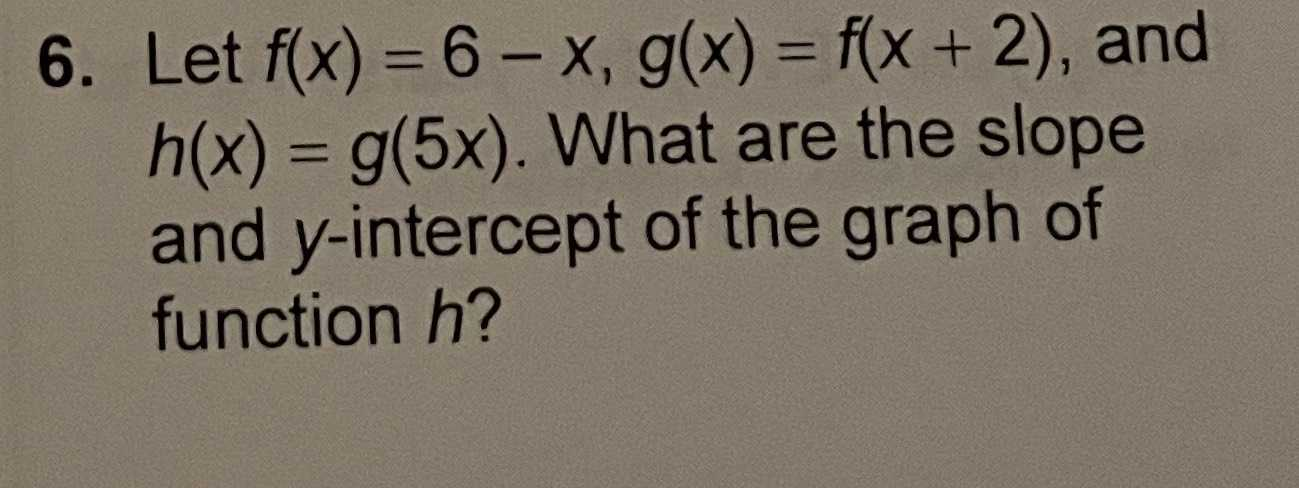### ¿Todavía tienes preguntas de matemáticas?

Pregunte a nuestros tutores expertos
Algebra
Pregunta6. Let $$f ( x ) = 6 - x , g ( x ) = f ( x + 2 )$$ , and $$h ( x ) = g ( 5 x )$$ . What are the slope and $$y$$ -intercept of the graph of function $$h$$ ?